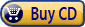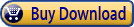Summary: Array formulas allow you to accomplish amazing things with your data, including things you cannot do with regular formulas. Here's the table of contents for this definitive guide on how you can put array formulas to work for you.

## Introduction

• Can't All this Stuff Be Done with Macros?
• Need More Tips?
• Sharing this Document

## Array Formula Basics

• Understanding Array Formulas
• Single-Cell Array Formulas
• Multi-Cell Array Formulas
• Some Array Formula Gotchas
• Analyzing an Array Formula
• Editing Multi-Cell Array Formulas
• Copying an Array Formula
• Easily Selecting an Array Formula Range
• Array Formulas without Cells
• Checking for Proper Entry of Array Formulas
• Stopping the Deletion of Cells

## Using Array Formulas to Count

• Counting with Two Criteria
• Counting Records Matching Multiple Criteria
• Counting Dates in a Range
• Counting Unique Values with Functions
• Counting Unique Values
• Counting Cells with Specific Characters
• Counting Groupings Below a Threshold
• Counting Commas in a Selection
• Counting Wins and Losses
• Getting a Count of Unique Names
• Counting Asterisks
• Counting Asterisks in a Column
• Counting Odds and Evens
• Finding Odd Values Greater Than 50
• Getting a Conditional Count of Cells Containing Values

## Using Array Formulas with Numbers

• Summing Digits in a Value
• Summing Absolute Values
• Summing Every Fourth Cell in a Row
• Summing Based on Part of the Information in a Cell
• Using a Numeric Portion of a Cell in a Formula
• Calculating the Median Age of a Group of People
• An Average that Excludes Zero Values
• Deriving High and Low Non-Zero Values
• Median of Selected Numbers
• Calculating a Geometric Standard Deviation
• Using GEOMEAN With a Large List
• Excluding Values from Averaging
• Finding the Smallest Even Value
• Returning the Smallest Non-Zero Value
• Last Non-Zero Value in a Row
• Returning the Minimum of Integers of a Range
• Extracting Numbers within a Range
• Picking Different Random Numbers from a Range
• Identifying Missing Numbers in a Consecutive Series
• Separating Evens and Odds

## Using Array Formulas with Text

• Comparing Lists for Duplicates
• Finding the First Non-Digit in a Text Value
• Inserting Dashes between Letters and Numbers
• Getting Rid of Everything Except Numbers
• Breaking Up Variable-Length Part Numbers
• Returning Item Codes Instead of Item Names
• Compiling a List of Students in a Course
• Splitting Cells by Case
• Checking for Messages in Cells
• Deleting Duplicate Columns
• Checking for Either of Two Text Values
• Extracting Street Numbers from an Address

## Using Array Formulas with Dates

• Weekdays in a Month
• Finding the Date Associated with a Negative Value
• Years in Which a Date Occurred on a Particular Day
• Determining a Name for a Week Number
• Calculating Averages by Date
• Averaging Values for a Given Month and Year
• Deriving Monthly Median Values
• Counting Jobs Completed On a Date
• Getting Rid of All Rows Except the One for the Latest Date
• Creating a Year-to-Date Comparison Chart

## Using Array Formulas for Lookups

• Complex Lookup Formulas
• Making VLOOKUP Case Sensitive
• Looking Backward through a Data Table
• Locating a Single-Occurrence Value in a Column
• Pulling a Phone Number with a Known First and Last Name
• Searching for a Value Using a Function
To celebrate the release of the fifth edition of ExcelTips: Amazing Array Formulas, for a limited time (through December 12, 2018) you can download your own copy of this valuable e-book for 30% off the regular price. Get your discount; place your order today!

ExcelTips: Amazing Array Formulas